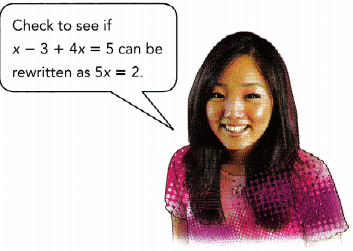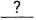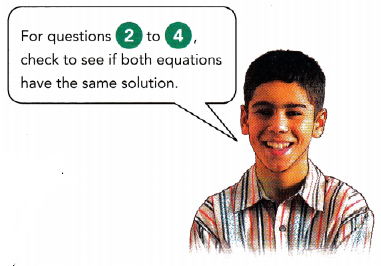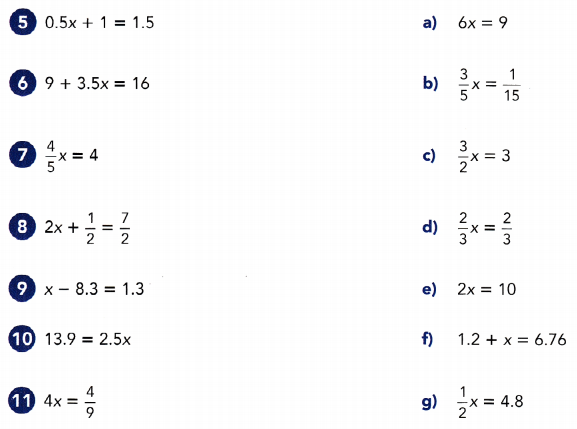# Math in Focus Grade 7 Chapter 4 Lesson 4.1 Answer Key Understanding Equivalent Equations

Practice the problems of Math in Focus Grade 7 Workbook Answer Key Chapter 4 Lesson 4.1 Understanding Equivalent Equations to score better marks in the exam.

## Math in Focus Grade 7 Course 2 A Chapter 4 Lesson 4.1 Answer Key Understanding Equivalent Equations

### Math in Focus Grade 7 Chapter 4 Lesson 4.1 Guided Practice Answer Key

Copy and complete to state whether each pair of equations are equivalent equations. Give a reason for your answer.

Question 1.x – 3 + 4x = 5 and 5x = 2
x – 3 + 4x = 5
5x – 3 = 5 Group like terms.
5x – 3 += 5 +Addto both sides.
5x =Simplify.
x – 3 + 4x = 5be rewritten as 5x = 2.
So, the equations havesolutions and areNo, they are not equivalent equations.

Explanation:
Given 1st equation, x – 3 + 4x = 5

It can also be written as 5x – 3 =5

Add 3 to both sides, 5x – 3 + 3 = 5 + 3

5x = 8

So, equation x – 3 + 4x = 5  cannot be written as 5x = 2Question 2.
x + 7 = 12 and 2x = 10
First solve x + 7 = 12.
x + 7 = 12
x + 7 –= 12 –Subtractfrom both sides.
x =Simplify.
Then check to see ifis the solution of the equation 2x = 10.
If x =, 2x = 2 •Substitutefor x.
=a solution.
Because the equations have thesolution, they areequations.
Yes, Both are equivalent equations.

Explanation:
First, let’s solve x + 7 = 12

Subtract 7 on both sides.

x + 7 – 7 = 12 -7

x = 5

Lets consider second equation 2x = 10

substitute x= 5 which we got by solving  the 1st equation in the 2nd equation.

2.(5) = 10

Hence proved.

Question 3.
1.2x = 2.4 and x – 6 = 8
First solve x – 6 = 8.
x – 6 = 8
x – 6 += 8 +Addto both sides,
x =Simplify.
Then check to see ifis the solution of the equation 1.2x = 2.4.
If x =, 1.2x = 1.2 •Substitutefor x.
=a solution.
Because the equations havesolutions, they areequations.
No, they are not equivalent equations.

Explanation:
First, lets consider x – 6 = 8

x – 6 + 6 = 8 + 6

x = 14

Lets substitute x = 14  in the first equation.

1.2 x = 2.4

1.2(14) = 16.8

Both the equations are not equivalent equations.

Question 4.
$$\frac{2}{5}$$x = 4 and x = 10.
If x = 10, $$\frac{2}{5}$$x = $$\frac{2}{5}$$ • 10 Substitute 10 for x.
=a solution.
Because the equations have thesolution, they areequations.
Yes, both the equations have the same solution, they are equivalent equations.

Explanation:
Let’s consider first equation, (2/5)x =4

Let us  substitute the second equation, x =10 in first equation.

(2/5) × 10 = 4

Hence proved.

Both the equations have the same solution, they are equivalent equations.

### Math in Focus Course 2A Practice 4.1 Answer Key

Tell whether each pair of equations are equivalent. Give a reason for your answer.

Question 1.
2x = 4 and 4x + 5 = 13
Yes, Both the equations are equivalent.

Explanation:
Let us consider second equation, 4x + 5 = 13

Subtract 5 on both sides.

4x + 5 – 5 = 13 – 5

4x = 8

x = 8 ÷ 4

x = 2

Substitute x=2 in the first equation, 2x = 4

2(2) = 4

Hence proved.

Both the equations are equivalent.

Question 2.
-2x + 9 = 7 and —2x = 2
No, both the equations are not equivalent.

Explanation:
Lets solve the first equation, -2x + 9 = 7

Subtract 9  on both sides.

-2x + 9 – 9 = 7 – 9

-2x = -2

The second equation is -2x = 2

So, both the equations are not equivalent.

Question 3.
5x – 4 + 3x = 8 and 8x = 12
Both are equivalent equations.

Explanation:
Lets us consider first equation, 5x – 4 + 3x = 8

8x – 4 = 8

8x = 8 + 4

8x = 12

As we derived second equation from the first equation, both are equivalent equations.

Question 4.
$$\frac{3}{4}$$x – 7 = 2 and x = 12
Yes, both are equivalent equations.

Explanation:
Let us consider first equation, (3/4)x = 2

Substitute, the second equation, x = 12 in the first equation.

(3/4)(12) – 7 = (3).(3) – 7

=9 – 7

=2

Hence proved. Both are equivalent equations.

Match each equation with an equivalent equation.5 – d
6 – c
7 – e
8 – a
9 – g
10 – f
11 – b

Explanation:
5.  0.5x + 1 = 1.5

0.5x = 1.5 – 1 = 0.5

x = 0.5 ÷ 0.5

x = 1

We can match 5th equation with equation (d) on the other side.

6. 9 + 3.5x = 16

Let us solve the equation

3.5x = 16 – 9

3.5x = 7

x = 7 ÷ 3.5

x = 2

We can match the equation (3/2)x = 3

x = 3 × (2/3)

x = 2

Hence both are same.

7. (4/5)x =4

4x = 20

x =5

We can match 7th equation with equation (e) on the other side.

2x =10

x =5

Hence Matched.

8.  2x + (1/2) = (7/2)

2x = (7/2) – (1/2)

2x = (6/2)

2x = 3

x = 3/2

As option (a) is 6x =9

It can be also written as 3/2 as both are dividends of 3.

9. x – 8.3 = 1.3

x = 1.3 + 8.3

x = 9.6

Option (g) is  (1/2)x = 4.8

x =9.6

Hence matched.

10. 13.9 = 2.5x

x = 13.9 ÷ 2.5

x = 5.56

Option (f) is 1.2 + x = 6.76

x = 6.76 – 1.2

x =5.56

Hence proved.

11. 4x = (4/9)

x = 1/9

We can match with option (b).

(3/5)x = (1/15)

x = (1/9)

Solve.

Question 12.
Math Journal Max was asked to write to $$\frac{2}{3}$$x = 3 – x. He wrote the following:
$$\frac{2}{3}$$x = 3 – x
$$\frac{2}{3}$$x ∙ 3 = 3 ∙ 3 – x
2x = 9 – x
He concluded that $$\frac{2}{3}$$x = 3 – x and 2x = 9 $$\frac{2}{3}$$ x are equivalent equations. Do you agree with his conclusion? Give a reason for your answer.
No, I don’t agree with Max, both are not equivalent equations.

Explanation:
Let us consider first equation, (2/3)x = 3 – x

2x = 9 -3x (Max gave the equation 2x = 9 – x which is wrong, if we multiplied 3 on both sides not only it is multiplied with 3 it is also multiplies with x. so it is 2x = 9 -3x)

5x = 9

x = 9/5

x = 1.8

The second equation, 9(2/3)x =2x

substitute x =1.8 in the second equation

2 (1.8) = 9(2/3)(1.8)

3.6 = 10.8

Hence the both the equations are not equivalent.